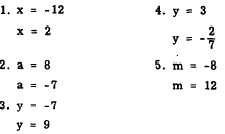Custom SearchSOLUTION BY FACTORING The equation x2 - 36 = 0 is a pure quadratic equation, There are two numbers which, when substituted for x, will satisfy the equation as follows: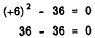also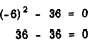Thus, +6 and - 6 are roots of the equation         x2 - 36 = 0 The most direct way to solve a pure quadratic (one in which no x term appears and the constant term is a perfect square) involves rewriting with the constant term in the right member, as follows:         X2 = 36 Taking square roots on both sides, we have         x = �6 The reason for expressing the solution as both plus and minus 6 is found in the fact that both +6 and -6, when squared, produce 36. The equation         x2 - 36 = 0 can also be solved by factoring, as follows: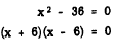We now have the product of two factors equal to zero. According to the zero factor law, if a product is zero, then one or more of its factors is zero. Therefore, at least one of the factors must be zero, and it makes no difference which one. We are free to set first one factor and then the other factor equal to zero. In so doing we derive two solutions or roots of the equation. If x + 6 is the factor whose value is 0, then we haveIf x - 6 is the zero factor, we have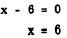When a three-term quadratic is put into simplest form, it is customary to place all the terms on the left side of the equality sign with the squared term first, the-first-degree term next, and the constant term last, as in         9x2 - 2x+7=0 If the trinomial in the left member is readily factorable, the equation can be solved quickly by separating the trinomial into factors. Consider the equation         3x2 - x - 2 = 0 By factoring the trinomial, the equation becomes         (3x + 2)(x - 1) = 0 Once again we have two factors, the product of which is 0. This means that one or the other of them (or both) must have the value 0. If the zero factor is 3x + 2, we have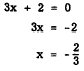If the zero factor is x - 1, we have         x - 1 = 0         x = 1 Substituting first x = 1 and then x = -2/3 in the original equation, we see that both roots satisfy it. Thus,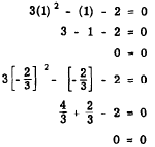In summation, when a quadratic may be readily factored, the process for finding its roots is as follows:. 1. Arrange the equation in the order of the descending powers of the variable so that all the terms appear in the left member and zero appears in the right. 2. Factor the left member of the equation. 3. Set each factor containing the variable equal to zero and solve the resulting equations. 4. Check by substituting each of the derived roots in the original equation. EXAMPLE: Solve the equation x2 - 4x = 12 for x.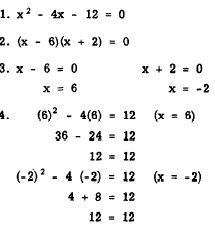Practice problems. Solve the following equations by factoring:Answers: